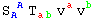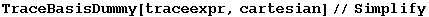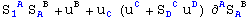5. Tracing contractions

One of the most important functions of xCoba` is TraceBasisDummy. We already know how to express a tensor on a given basis, as a contraction with Basis objects. With this new function, we can expand that contraction as a sum of coordinate components.

TraceBasisDummy            Expand dummy basis indices into their coordinate ranges

Tracing basis contractions.

In:=Out=A shorthand is provided. v[{a,cart}]◦v[{a,cart}], where ◦ is SmallCircle ( :sc:), has the same effect

In:=Out=Again, there is complete control over which dummies should be expanded. The complete syntax is TraceBasisDummy[expr, indices], where indices is one of the following:
- A single BIndex.
- A list of basis indices with head Indices.
- A vbundle (all basis dummies belonging to it will we expanded).
- A basis

In:=Out=In:=Out=In:=Out=In:=Out=In:=Out=With derivatives,

In:=Out=In:=Out=In:=Out=In:=Out=Another example

In:=Out=In:=Out=Created by Mathematica  (May 16, 2008)